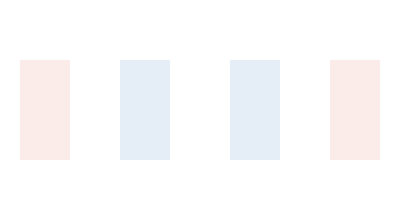# context.scale()

## Overview

The `context.scale()` method applies scale factors to the context's coordinate system, making the size of drawings larger or smaller. The coordinate system can be scaled in the x- and y-directions independently by passing it two values:

``context.scale(x, y);``

The `x` value scales the coordinate system in the x-direction and the `y` value scales it in the y-direction. For example, `context.scale(2.0, 0.5)` scales the coordinate system so new shapes are drawn twice as wide and half as tall. This only applies to shapes drawn after the coordinate system has been scaled. Any shapes drawn on the canvas before the coordinate system is scaled are unaffected.

## Scale a Drawing

In this example, we scale the context's coordinate system and then draw a tree.

We start by scaling the coordinate system by scale factors of 1 in both the x- and y-directions. This does nothing for now, which is what we want. We'll scale the coordinate system later.

``context.scale(1, 1); // Apply scale factors to the coordinate system here``

Then, we draw a tree:

``````context.fillStyle = 'Sienna';
context.fillRect(110, 40, 20, 100); // Draw tree trunk
context.fillStyle = 'ForestGreen';
context.beginPath();
context.arc(120, 40, 30, 0, 2 * Math.PI, false);
context.fill(); // Draw leaves``````

At this point, we have drawn the tree with the coordinate system at normal scale.

Now, go back up to the top of the program where we set scale factors for the coordinate system and change the `x` scale factor to 3.0 and the `y` scale factor to 1.5. When we draw the tree in this new coordinate system, it will be three times as wide and 1.5 times as tall on the canvas.

``context.scale(3.0, 1.5);``

Change the values of the scale factors for the coordinate system to see what happens. To learn more about the coordinate system, visit the Coordinates lesson.

Quick Reference:

Message Log

## Challenge 1

Use the `context.scale()` method to apply scale factors to the context's coordinate system so the French flag is 2.5 times as wide and 2.5 times as tall.

Message Log
##### Canvas (your drawing will display here)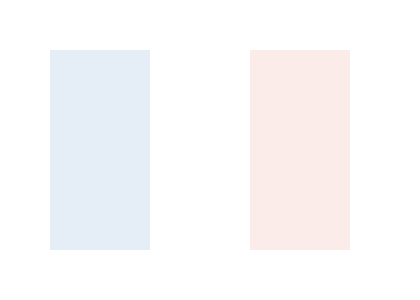## Scale Multiple Drawings

When we use the `context.scale()` method to scale a drawing, it is important to remember that we are scaling the entire coordinate system, not just a single drawing.

In this example, we scale a row of trees.

Since we are drawing more than one tree, we start by defining the `drawTree()` function, which has parameters for the x- and y-coordinates of the tree.

``````function drawTree(x, y) {
context.fillStyle = 'Sienna';
context.fillRect(x - 10, y, 20, 100);
context.fillStyle = 'ForestGreen';
context.beginPath();
context.arc(x, y, 30, 0, 2 * Math.PI, false);
context.fill();
}``````

Then, we set the scale factors for the coordinate system and draw four trees each 80 pixels apart:

``````context.scale(1, 1);

drawTree(20, 40);
drawTree(100, 40);
drawTree(180, 40);
drawTree(260, 40);``````

Change the scale factors of the coordinate system to other values to see what happens. Not only are the trees scaled, but the distance between the trees are scaled as well.

Quick Reference:

Message Log

## Challenge 2

Use the `context.scale()` method to apply scale factors to the coordinate system so each French flag is drawn 1.4 times as tall.

Then, use the `drawFrenchFlag()` function to draw four French flags at the coordinates (40, 20), (240, 20), (60, 120), and (260, 120).

What value can be used for the `x` scale factor in the `context.scale()` method so the widths of the French flags are not scaled?

Message Log
##### Canvas (your drawing will display here)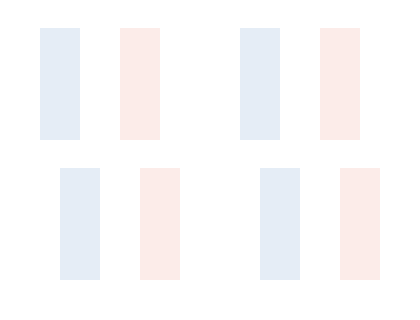## Combine Multiple Scale Factors

In this example, we draw three trees.

We draw the first tree at normal scale:

``drawTree(140, 40);``

We use the `context.scale()` method to scale the coordinate system by a scale factor of 1.5 in both the x- and y-directions and draw a second tree:

``````context.scale(1.5, 1.5);
drawTree(140, 40);``````

Finally, we use the `context.scale()` method to scale the coordinate system again by another scale factor of 1.5 in both the x- and y-directions and draw a third tree:

``````context.scale(1.5, 1.5);
drawTree(140, 40);``````

Note that the third tree isn't 1.5 times as large as the original tree. It is 1.5 times as large as the second tree and 2.25 times as large as the original tree. Because the `context.scale()` method scales the entire coordinate system, the scale factors multiply when more than one is applied (1.5 x 1.5 = 2.25).

Quick Reference:

Message Log

## Challenge 3

The program below draws three rectangles that are all the same size.

Use the `context.scale()` method to scale the coordinate system to draw the second rectangle twice as wide and three times as tall as the first rectangle.

Then, use the `context.scale()` method again to scale the coordinate system to draw the third rectangle four times as wide and 1.5 times as tall as the first rectangle.

Note the third rectangle is four times as wide and 1.5 times as tall as the first rectangle, not the second rectangle. Figure out scale factors to make this work.

Message Log
##### Canvas (your drawing will display here)## Restore the Coordinate System After Scaling

Sometimes we want to combine our scale factors, like when we are drawing a row of trees getting closer and closer. But usually, we want to restore the coordinate system after we scale it. Because the transformation matrix for the coordinate system is stored in the canvas's drawing state, we can use the `context.save()` and `context.restore()` methods to save the coordinate system and restore it back to its original state.

In this example, we start by saving the drawing state when the scale of the coordinate system is normal:

``context.save(); // Save the drawing state when the scale is normal``

Then, we change the scale of the coordinate system, set the `context.fillStyle` property to the color `'OrangeRed'`, and draw a line of text:

``````context.save(); // Save the drawing state when the scale is normal
context.scale(2, 2);
context.fillStyle = 'OrangeRed';
context.fillText('scale(2, 2)', 10, 10);
context.restore(); // Restore the drawing state back to when the scale is normal``````

Before changing the scale of the coordinate system again and drawing a second line of text, we restore the drawing state we saved earlier. This restores the scale of the coordinate system back to normal. Now when we scale the coordinate system two more times to draw the second and third lines of text, the scale factors aren't multiplied together because we restore the coordinate system between transformations.

It's important to note that we need to save the drawing state again immediately after restoring it if we want to return to the same drawing state again later. To learn more about saving and restoring the drawing state, visit the save()/restore() lesson.

Quick Reference:

Message Log

## Challenge 4

The `drawTree()` function is used to draw four trees in the program below.

Update the program so the first tree is drawn at normal scale, but the second, third, and fourth trees are drawn 1.3, 0.8, and 1.2 times as tall as the first tree, respectively.

Between transformations, use the `context.save()` and `context.restore()` methods to save and restore the drawing state.

Message Log
##### Canvas (your drawing will display here)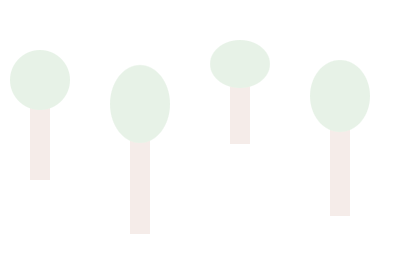## Use Translation to Anchor a Scaled Drawing

When scaling the trees in the previous challenge, the tops and bottoms of the trees were no longer lined up even though all four trees were positioned at the same y-coordinate. This is because the `context.scale()` method applies its scale factors to the coordinate system, not individual drawings.

When the coordinate system is scaled, every point in the coordinate system moves either closer to or farther away from the origin, except the origin itself. Since the origin doesn't move, it acts as a kind of anchor point.

In this example, we draw two trees. One tree is drawn while the origin of the coordinate system is in the top left corner of the canvas. This is the origin's default position. Before drawing the other tree, we move the origin to the base of the tree using the `context.translate()` method. Because the origin of the coordinate system is positioned at the base of the tree, the base of the tree does not move when the coordinate system is scaled.

We start by modifying the `drawTree()` function so the bottom center point of the tree is now drawn at the `x` and `y` values passed into the function.

``````function drawTree(x, y) {
context.fillStyle = 'Sienna';
context.fillRect(x - 10, y - 100, 20, 100);
context.fillStyle = 'ForestGreen';
context.beginPath();
context.arc(x, y - 100, 30, 0, 2 * Math.PI, false);
context.fill();
}``````

We position the first tree normally. The origin of the coordinate system is in the top left corner of the canvas and the bottom center point of the tree is positioned at (60, 150), which is the point 60 pixels to the right of the origin and 150 pixels down.

``````context.save();
context.scale(1, 1); // Scale the coordinate system to draw the first tree
drawTree(60, 150); // Draw the first tree so its bottom center point is at (60, 150)
context.restore();``````

Try changing the scale of the coordinate system before the first tree is drawn. Increasing the scale moves the tree farther from the origin. Decreasing the scale moves it closer.

Instead of positioning the second tree by passing in `x` and `y` values, we use the `context.translate()` method to move the origin of the coordinate system to where we want to draw the tree. By moving the origin, we can actually position the bottom center point of the tree at the origin (0, 0).

``````context.save();
context.translate(320, 280); // Move the origin of the coordinate system to (320, 280)
context.scale(1, 1); // Scale the coordinate system to draw the second tree
drawTree(0, 0); // Draw the second tree so its bottom center point is at the origin (0, 0)
context.restore();``````

Try changing the scale of the coordinate system before the second tree is drawn. Because the bottom center point of the tree is at the origin, the bottom center point of the tree is anchored and does not move.

Quick Reference:

Message Log

## Challenge 5

The program below draws three blue frames that are all the same size. The first frame is positioned at the coordinates (20, 20), the second at (180, 80), and the third at (60, 140).

Use the `context.scale()` method to scale each frame so the first frame is 4 times wider and 3 times taller, the second frame is 3 times wider and 3.5 times taller, and the third frame is 4 times wider and 2 times taller.

However, before scaling the frames, use the `context.translate()` method to anchor the top left corner of each frame at the origin of the coordinate system so the top left corner of the frame doesn't move when the coordinate system is scaled. This means that, instead of using `drawFrame(20, 20, 'MediumBlue')` to position the first frame, position the frame at (20, 20) using the `context.translate()` method and then draw the frame using `drawFrame(0, 0, 'MediumBlue')`.

Remember to save and restore the drawing state before and after translating and scaling the coordinate system and drawing each frame.

Message Log
##### Canvas (your drawing will display here)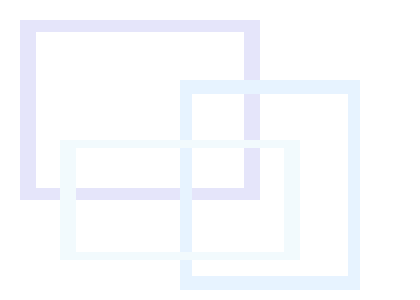## Create a Vanishing Point

In this example, we use the `context.translate()` and `context.scale()` methods to create a vanishing point on the horizon to give our drawing a 3D effect.

If we use the `context.scale()` method to scale the coordinate system down, shapes will be drawn smaller and closer to the origin. If we keep scaling the coordinate system down, those shapes will eventually vanish into the origin.

We start by using the `context.translate()` method to position the origin of the coordinate system. The position of the origin will be the position of our vanishing point.

``````var x = 240;
var y = 280; // Coordinates of the vanishing point

context.save() // Save the original drawing state
context.translate(x, y); // Move the origin to the vanishing point on the canvas``````

To make the vanishing point and horizon easier to see, we draw a line at the horizon and a dot at the vanishing point:

``````drawHorizon();
drawVanishingPoint();``````

Then, we set up a for loop to repeatedly scale the coordinate system and draw two squares. The for loop runs through the loop eight times.

``````for (var i = 0; i < 8; i = i + 1) {
context.scale(1.26, 1.26);
drawSquare(5, -40); // The square to the right of the vanishing point
drawSquare(-40, -30); // The square to the left of the vanishing point
}``````

Each time through the for loop, we scale the coordinate system using a scale factor of 1.26 in both the x- and y-directions. Because we are not saving and restoring the drawing state between transformations, our scale factors are combining, which is what we want in this situation. We want to keep scaling the coordinate system so the drawings get larnger and larger.

Finally, we use the `drawSquare()` function to draw two squares, both with a width and height of 20, filled with the color `'DarkOrange'`, and outlined in the color `'DarkRed'`. The first square is positioned at (-40, -30) and the second square is positioned at (5, -40).

Note that the two squares are drawn at the same positions for each loop. Because we are scaling the coordinate systems, the squares appear to be moving toward us from the vanishing point. Change the positions of the vanishing point and the two squares in the for loop to see what happens.

Quick Reference:

Message Log

## Challenge 6

Update the program below to draw six trees so they appear to moving toward us from a vanishing point.

Position the vanishing point at the coordinates (-80, 140). Note that this point is outside the left edge of the canvas, so we want actually see it on the canvas.

Use the `drawTree()` function to draw the trees at position (120, 45). Before drawing a tree, scale the coordinate system by a scale factor of 1.2 in both the x- and y-directions. Allow the scale factors to multiply.

Message Log
##### Canvas (your drawing will display here)## Create a Mirror Image

In this example, we use the `context.scale()` method to create a mirror image of a line of text by reflecting it over the coordinate system's x-axis.

We start by using the `context.translate()` method to move the origin of the coordinate system to (100, 150). The position of the origin is important because, when we reflect the line of text, it will be reflected over the x-axis. This means the x-axis will be our line of symmetry.

``````var x = 100;
var y = 150; // Coordinates of the origin

context.save(); // Save the original drawing state
context.translate(x, y); // Move the origin``````

To make the line of symmetry easier to see, we draw lines on the x- and y-axes:

``drawAxes();``

After setting the `context.fillStyle`, `context.font`, and `context.textBaseline` properties, we draw the original line of text at (20, -180):

``````context.fillStyle = 'Crimson';
context.font = '48px Arial';
context.textBaseline = 'top';

context.fillText('Mirror Image', 20, -80); // Original line of text``````

Because the origin of the coordinate system is at (100, 150) on the canvas, positioning the line of text at (20, -80) on the coordinate system actually puts the top left corner of the text at (120, 70) on the canvas.

To create a mirror image of the line of text, we use the `context.scale()` method to reflect the entire coordinate system over the x-axis by using a scale factor of -1 in the y-direction. This reverses the direction of the y-axis, so up is down and down is up. To reflect a drawing horizontally over the y-axis, we would use a scale factor of -1 in the x-direction. Then, we draw the same line of text at the exact same position on the coordinate system.

``````context.scale(1, -1);
context.fillText('Mirror Image', 20, -80); // Reflected line of text``````

Because the origin of the coordinate system is at (100, 150) on the canvas and we reflected the coordinate system over the x-axis, positioning the line of text at (20, -80) on the coordinate system actually puts the bottom left corner of the text at (120, 230) on the canvas.

Try changing the scale factor in the x-direction to -1 to see what happens. We can also reflect and scale drawings by using negative values greater or less than -1.

Quick Reference:

Message Log

## Challenge 7

Draw two French flags using the `drawFrenchFlag()` function, which accepts `x` and `y` values for its coordinates. Position the original French flag so its top left corner is at (230, 60) on the canvas. Then, reflect the second French flag over the y-axis and position it so its top right corner is at (170, 60).